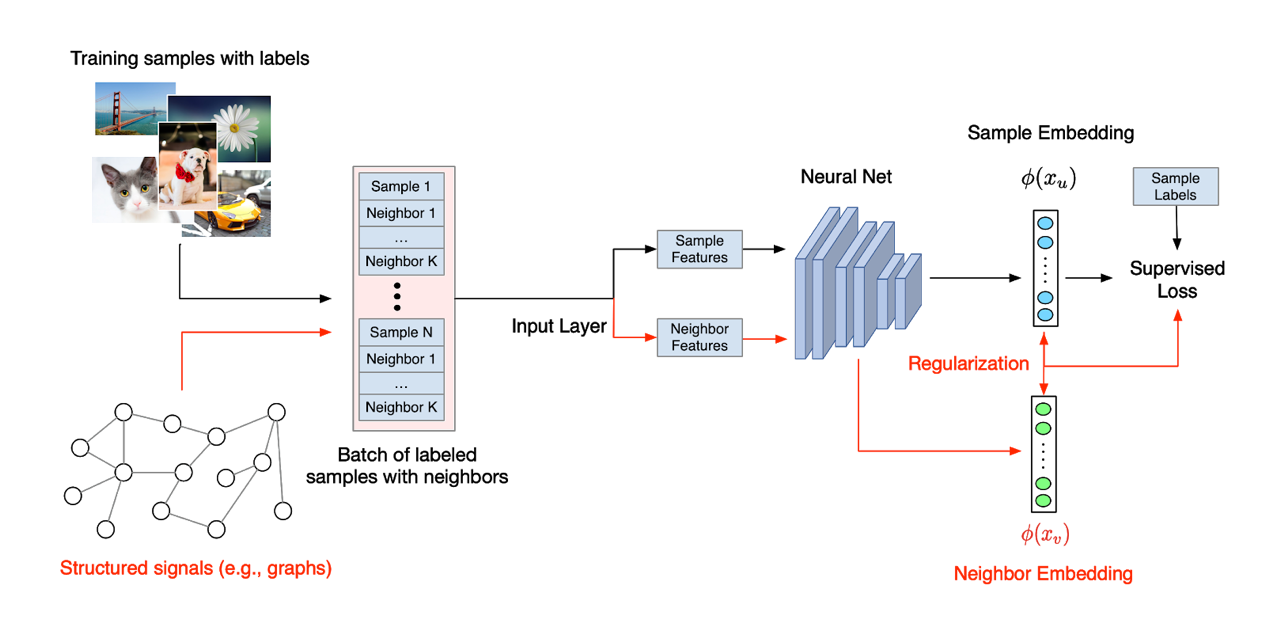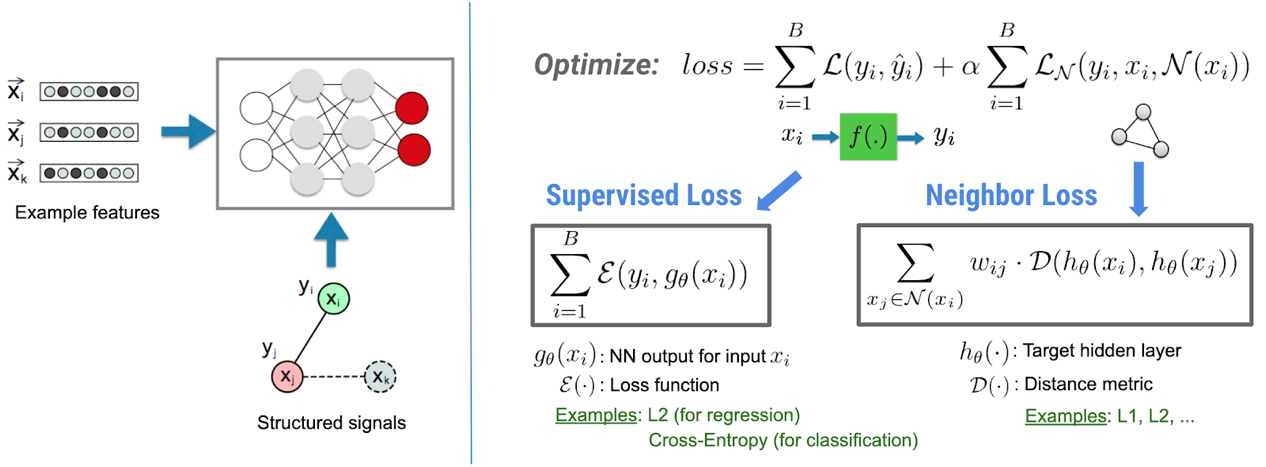# 谷歌开源基于 TensorFlow 的神经结构学习框架 NSL，可用于图数据训练NSL 帮助 TensorFlow 用户轻松将各类结构化信号结合起来，共同用于训练神经网络，同时适用于多种不同的学习场景，包括监督、半监督与无监督（表示）等设置。

## 神经结构学习（NSL）的工作原理## 利用神经结构学习（NSL）创建模型

python pack_nbrs.py --max_nbrs=5 \labeled_data.tfr \unlabeled_data.tfr \graph.tsv \merged_examples.tfr 

import neural_structured_learning as nsl# Create a custom model — sequential, functional, or subclass. base_model = tf.keras.Sequential(…)# Wrap the custom model with graph regularization. graph_config = nsl.configs.GraphRegConfig( neighbor_config=nsl.configs.GraphNeighborConfig(max_neighbors=1)) graph_model = nsl.keras.GraphRegularization(base_model, graph_config)# Compile, train, and evaluate. graph_model.compile(optimizer=’adam’,  loss=tf.keras.losses.SparseCategoricalCrossentropy(), metrics=[‘accuracy’]) graph_model.fit(train_dataset, epochs=5) graph_model.evaluate(test_dataset)

## 如果没有显式结构，该怎么办？

import neural_structured_learning as nsl# Create a base model — sequential, functional, or subclass. model = tf.keras.Sequential(…)# Wrap the model with adversarial regularization. adv_config = nsl.configs.make_adv_reg_config(multiplier=0.2, adv_step_size=0.05) adv_model = nsl.keras.AdversarialRegularization(model, adv_config)# Compile, train, and evaluate. adv_model.compile(optimizer=’adam’, loss=’sparse_categorical_crossentropy’, metrics=[‘accuracy’]) adv_model.fit({‘feature’: x_train, ‘label’: y_train}, epochs=5) adv_model.evaluate({‘feature’: x_test, ‘label’: y_test})

https://www.tensorflow.org/neural_structured_learning/